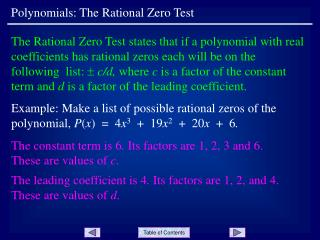Download PresentationPolynomials: The Rational Zero TestPolynomials: The Rational Zero Test - PowerPoint PPT Presentation

Download PresentationPolynomials: The Rational Zero Test
An Image/Link below is provided (as is) to download presentation

Download Policy: Content on the Website is provided to you AS IS for your information and personal use and may not be sold / licensed / shared on other websites without getting consent from its author. While downloading, if for some reason you are not able to download a presentation, the publisher may have deleted the file from their server.

- - - - - - - - - - - - - - - - - - - - - - - - - - - E N D - - - - - - - - - - - - - - - - - - - - - - - - - - -
Presentation Transcript

1. Polynomials: The Rational Zero Test The Rational Zero Test states that if a polynomial with real coefficients has rational zeros each will be on the following list:  c/d, where c is a factor of the constant term and d is a factor of the leading coefficient. Example: Make a list of possible rational zeros of the polynomial, P(x) = 4x3 + 19x2 + 20x + 6. The constant term is 6. Its factors are 1, 2, 3 and 6. These are values of c. The leading coefficient is 4. Its factors are 1, 2, and 4. These are values of d.

2. Simplifying and removing duplicate fractions results in: Polynomials: The Rational Zero Test c-values – 1, 2, 3 and 6 d-values – 1, 2 and 4 Now form all fractions of the form:  c/d: Slide 2

3. The polynomial in the example, (P(x) = 4x3 + 19x2 + 20x + 6) actually has three real zeros: Polynomials: The Rational Zero Test Two of the zeros are irrational; only one is rational (- 3/4). The Rational Zero Test makes no claims beyond those for rational zeros. It simply states that if any rational zeros exist, they will appear on the computed list. Indeed, - 3/4 was on the computed list! Slide 3

4. The possible rational zeros are: Polynomials: The Rational Zero Test Try: Make a list of possible rational zeros of the polynomial, P(x) = 5x3 – 14x2 + 3x + 4. Slide 4

5. Polynomials: The Rational Zero Test END OF PRESENTATION Click to rerun the slideshow.